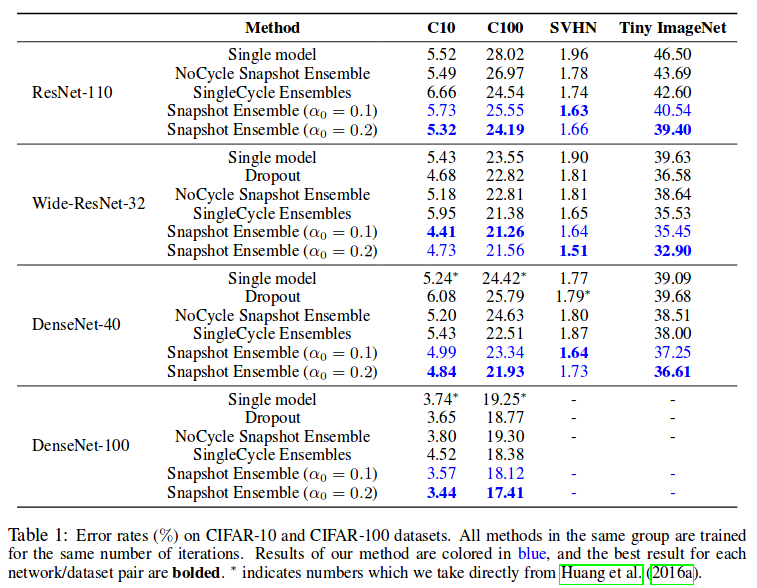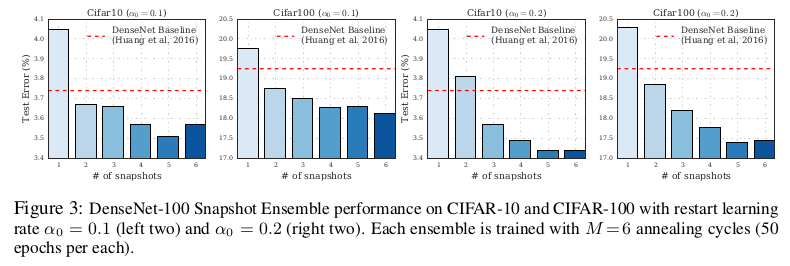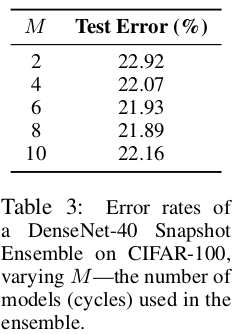### Description

In contrast to traditional ensembles (produce an ensemble of multiple neural networks), the goal of this work is training a single neural network, converging to several local minima along its optimization path and saving the model parameters to obtain a ensembles model. It is clear that the number of possible local minima grows exponentially with the number of parameters and different local minima often have very similar error rates, the corresponding neural networks tend to make different mistakes.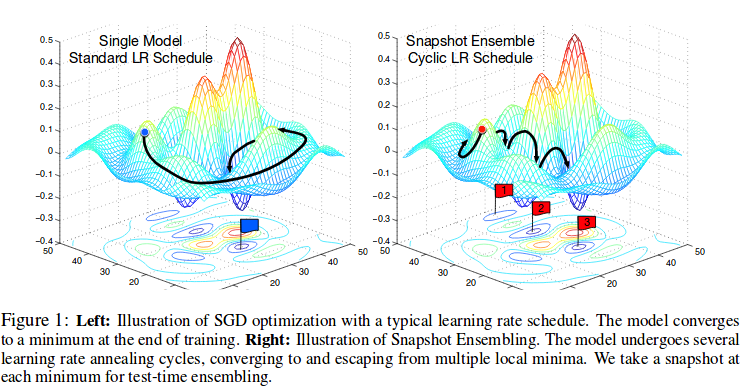Snapshot Ensembling generate an ensemble of accurate and diverse models from a single training with an optimization process which visits several local minima before converging to a final solution. In each local minima, they save the parameters as a model and then take model snapshots at these various minima, and average their predictions at test time.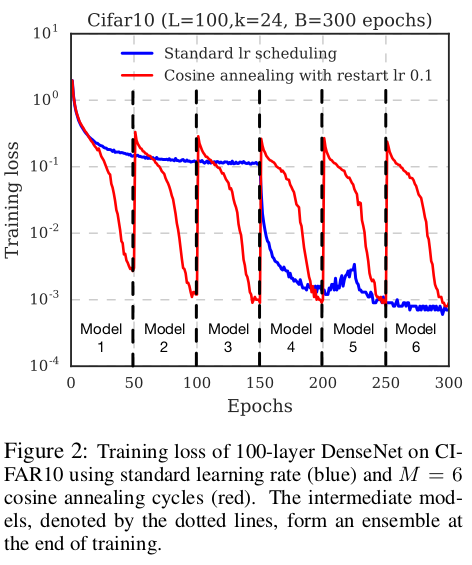They split the training process into “M” cycles, each of which starts with a large learning rate, which is connected to a smaller learning rate. They decrease the learning rate and encouraging the model to converge towards its first local minimum after as few as 50 epochs. The learning rate $\alpha$ has the form: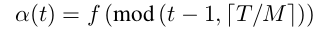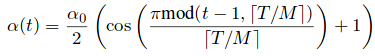where $t$ is the itiration number, $T$ is the total number of training iterations, anf $f$ is a monotonically decreaing function.

The large learning rate is $\alpha = f(0)$ and the small learning rate in last model is $\alpha = f(\frac{T}{M})$, drives it to a well local minimum point.

### Results:

Dataset : CIFAR, SVHN, Tiny ImageNet, ImageNet. Architectures: ResNet, Wide ResNet, DenseNet.